Electric Field

Electric Field

A charged particle exerts a force on particles around it. We can call the influence of this force on surroundings as electric field. It can be also stated as electrical force per charge. Electric field is represented with E and Newton per coulomb is the unit of it.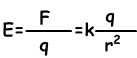Electric field is a vector quantity. And it decreases with the increasing distance.k=9.109Nm2/C2

• · Electric field cannot be seen, but you can observe the effects of it on charged particles inside electric field.
• · To find the electric field vector of a charge at one point, we assume that as if there is a +1 unit of charge there.
• · If you want to find the total electric field of the charges more than one, you should find them one by one and add them using vector quantities.

Electric Field Lines

Motion path of the “+” charge in an electric field is called electric field line. Intensity of the lines shows the intensity of the electric field. Pictures given below show the drawings of field line of the positive charge and negative charge.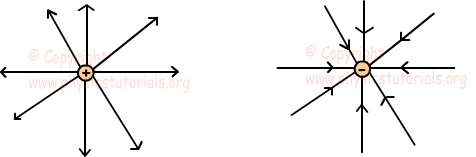Electric field lines;

• · Are perpendicular to the surfaces
• · Never intercept
• · If the electric field lines are parallel to each other, we call this regular electric field and it can be possible between two oppositely charged plates. E is constant within this plates and zero outside the plates.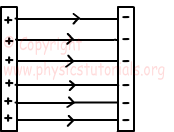• · We can find the E in these plates by connecting a power supply having potential difference V using following formula;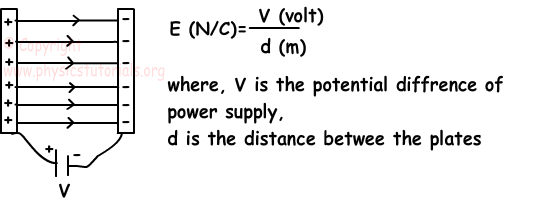Pictures given below show the path of lines of two same charges and two opposite charges.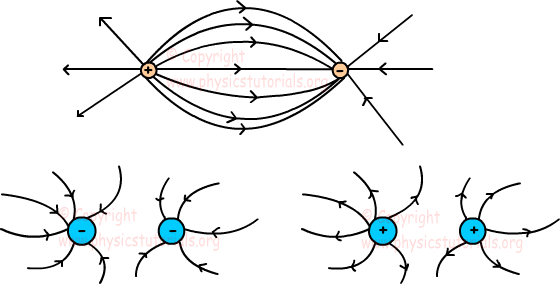Example: Find the electric field created by the charges A and B at point C in terms of k.q/d2?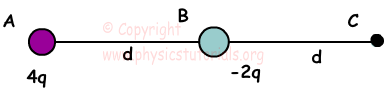Example: If the electric field at point A is zero, find the charge at point D in terms of q.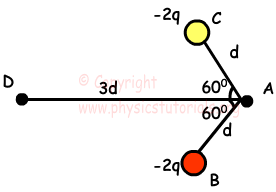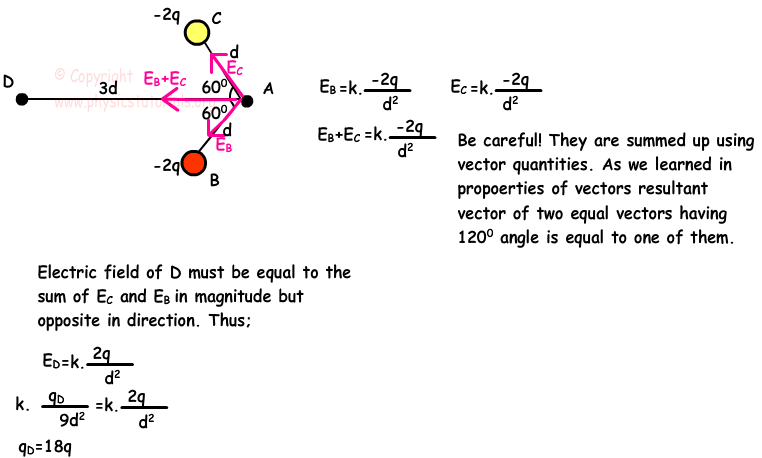Force Acting on a Charged Particle inside Electric Field

E=F/q

F=E.q where; F is the force acting on the charge inside the electric field E.Using this equation we can say that;

If q is positive then F=+E.q and directions of Force and Electric Field are same

If q is negative then F=-E.q and directions of Force and Electric Field are opposite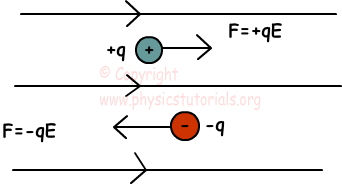Example: If the charge q having mass m is in equilibrium between the two plated having distance d, find the potential difference of power supply.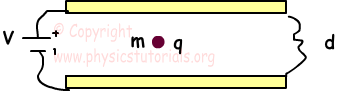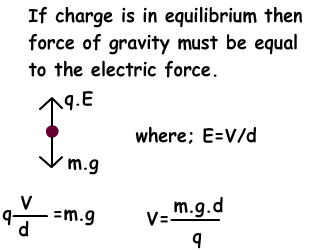Electric Field of a Conductor Sphere

There is a maximum electric field at surface of the sphere. As distance increases from the surface, electric field decreases. Finally, as it seen from the picture, inside the conductor sphere electric field is zero.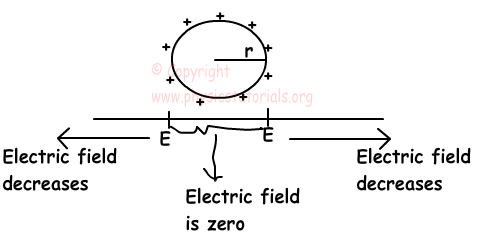Electrostatics Exams and Solutions

Author: